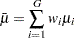### Analyses in the ONEWAYANOVA Statement

#### One-Degree-of-Freedom Contrast (TEST=CONTRAST)

The hypotheses are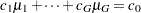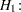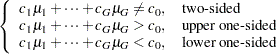where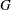is the number of groups,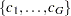are the contrast coefficients, andis the null contrast value.

The test is the usualtest for a contrast in one-way ANOVA. It assumes normal data with common group variances and requires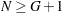and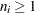.

O’Brien and Muller (1993, Section 8.2.3.2) give the exact power as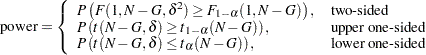where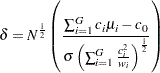#### Overall F Test (TEST=OVERALL)

The hypotheses are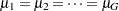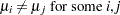whereis the number of groups.

The test is the usual overalltest for equality of means in one-way ANOVA. It assumes normal data with common group variances and requiresand.

O’Brien and Muller (1993, Section 8.2.3.1) give the exact power as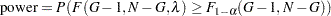where the noncentrality is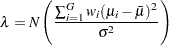and